nextnano.com  GUI: nextnanomat  Tool: nextnano++  Tool: nextnano³  Tool: nextnano.NEGF  Download | Search | Copyright | News | Publications  * password protected nextnano³ software2D QWR magnetic

# nextnano3 - Tutorial

## Hole wave functions in a quantum wire subjected to a magnetic field

Author: Stefan Birner

If you want to obtain the input files that are used within this tutorial, please contact stefan.birner@nextnano.de.
` -> 2Dwire.in                       ` (single-band approximation)```  -> 2Dwire_magnetic.in              ```(single-band approximation including magnetic field)```  -> 2Dwire_6x6kp.in                 ``` (6-band k.p approximation)```  -> 2Dwire_6x6kp_Burt.in            ``` (6-band k.p approximation)```  ```

## Quantum wire

Similar to the 1D confinement in a quantum well, it is possible to confine electrons or holes in two dimensions, i.e. in a quantum wire.
The quantum wire consists of InAs (blue area) and is confined by GaAs barriers (red area).
Its size is 10 nm x 10 nm whereas the whole simulation dimension is 30 nm x 30 nm.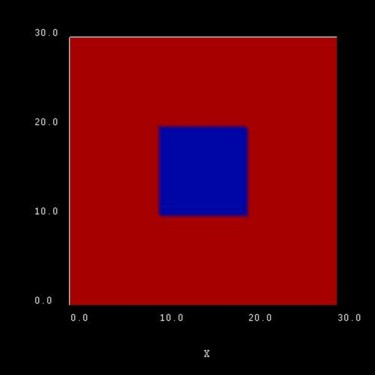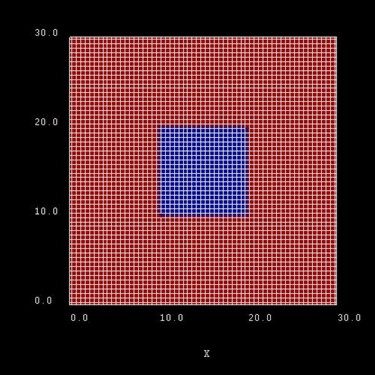The blue area is the quantum wire which consists of InAs. The quantum wire rectangle has the size 10 nm x 10 nm. This picture shows a possible configuration of rectangular grid lines. Here, the grid spacing is 0.5 nm, thus the quantum wire (blue area) consists of 21 x 21 = 400 grid points.

### Quantum cluster

Assumptions:
1) We apply Dirichlet boundary conditions to our quantum cluster (psi = 0 at the boundary).
2) The quantum cluster is defined only in the area of the quantum wire, i.e. from 10 nm to 20 nm.
These two conditions lead to an infinite GaAs barrier and thus the wave function is forced to be zero at the InAs quantum wire boundaries. Of course, this is not a realistic assumption but we simplify the sample to make the tutorial easier.

The energy levels and the wave functions of a rectangular quantum wire of length 10 nm with infinite barriers can be calculated analytically.

We assume that the barriers at the QD boundaries are infinite. This way we can compare our numerical calculations to analytical results.
The potential inside the quantum wire is assumed to be 0 eV.

As effective mass we take the isotropic heavy hole effective mass of InAs, i.e. mhh = 0.41 m0.
` valence-band-masses = 0.41d0   0.41d0   0.41d0  ! ` isotropic heavy hole effective```                        ...```

A discussion of the analytical solution of the 2D Schrödinger equation of a particle in a rectangle (i.e. quantum wire) with infinite barriers can be found in e.g.
Quantum Heterostructures (Microelectronics and Optoelectronics) by V.V. Mitin, V.A. Kochelap and M.A. Stroscio.

The solution of the Schrödinger equation leads to the following eigenvalues (where mhh is assumed to be negative):

En1,n2 = hbar2 pi2 / 2mhh                ( n12 / Lx2 + n22 / Ly2) =
= `-`9.1714667 * 10-19 eVm2 ( n12 / Lx2 + n22 / Ly2) =
= `-`0.0091714667 eV           ( n12           + n22       )           (if Lx = Ly = 10 nm)

• En1,n2 is the heavy hole energy in the two transverse directions, or the total heavy hole energy for kz = 0.
Note that the total heavy hole energy is given by Ehh = En1,n2 + hbar2 kz2 / 2mhh where kz is the wavevector along the z direction leading to a one-dimensional E(kz) dispersion.
• n1, n22 are two discrete quantum numbers (because we have two directions of quantization).
• Lx and Ly are the lengths along the x and y directions. In our case, Lx = Ly = 10 nm.

Generally, the energy levels are not degenerate, i.e. all energies are different.
However, some energy levels with different quantum numbers coincide, if the lengths along two directions are identical (En1,n2 = En2,n1) or
if their ratios are integers. In our quadratic quantum wire, the two lengths are identical.
Consequently, we expect the following degeneracies:

• E11 = `-`0.018343 eV (ground state)
• E12 = E21 = `-`0.045857 eV
• E22 = `-`0.073372 eV
• E13 = E31 = `-`0.091715 eV
• E23 = E32 = `-`0.119229 eV
• E14 = E41 = `-`0.155915 eV
• ...
• E18 = E81 = E47 = E74 = `-`0.596145 eV (Here, the degeneracy is a coincidence.)

nextnano³ numerical results for a 10 nm quadratic quantum wire with 0.10 nm grid spacing:

Output file name:``` Schroedinger_1band/ev2D_vb001_qc001_sg001_deg001_dir_Kx001_Ky001_Kz001.dat```

``` num_ev: eigenvalue [eV]:         (0.10 nm grid)   1      0.018341 ```= E11```   2     -0.045845  ```(two-fold degenerate)` `E12/E21```   3     -0.045845  ```(two-fold degenerate)` `E12/E21
```  4     -0.073348 ```= E22
```  5     -0.091653  ```(two-fold degenerate)` `E13/E31
```  6     -0.091653  ```(two-fold degenerate)` `E13/E31
```  7     -0.119156  ```(two-fold degenerate)` `E23/E32```   8     -0.119156  ```(two-fold degenerate)` `E23/E32
```  9     -0.155721  ```(two-fold degenerate)` `E14/E41
``` 10     -0.155721  ```(two-fold degenerate)` `E14/E41
` ...`
``` 42     -0.593061  ```(four-fold degenerate)` `E18/E81 or E47/E74
``` 43     -0.593061  ```(four-fold degenerate)` `E18/E81 or E47/E74```  44     -0.594144  ```(four-fold degenerate)` `E18/E81 or E47/E74
``` 45     -0.594144  ```(four-fold degenerate)` `E18/E81 or E47/E74

### Hole wave functions - Single-band effective-mass approximation (infinite GaAs barriers)

`-> 2Dwire.in`

The following figures show the charge densities (Psi²; Psi = hole wave function) of the four lowest energy confined hole eigenstates in an infinitely deep 10 nm x 10 nm InAs quantum wire. Due to the symmetry of the quantum wire, the second and the third eigenstate are degenerate.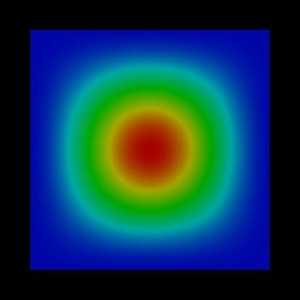1st eigenstate:` -0.0183 eV` 2nd eigenstate:` -0.0458 eV`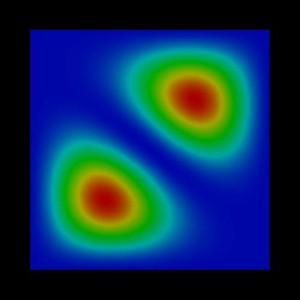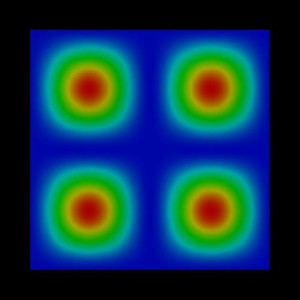3rd eigenstate:` -0.0458 eV` 4th eigenstate:` -0.0733 eV`

The heavy hole valence band edge energy of InAs is set to be located at 0 eV. The hole eigenvalues are:

 ```schroedinger-masses-anisotropic = yes``` ` = no` ` = box` `1st  eigenvalue  =>  ` confinement energy:` -0.018341 eV` ` -0.018341 eV` ` -0.018341 eV` `2nd  eigenvalue  =>  ` confinement energy:` -0.045845 eV` ` -0.045845 eV` ` -0.045845 eV` `3rd  eigenvalue  =>  ` confinement energy:` -0.045845 eV` ` -0.045845 eV` ` -0.045845 eV` `4th  eigenvalue  =>  ` confinement energy:` -0.073348 eV` ` -0.073348 eV` ` -0.073348 eV`

Note that these wave functions were obtained by using a single-band effective mass approximation for the holes. A more accurate and more realistic treatmeant would have been to use 6-band k.p. Note that the wire has been assumed to be unstrained (which is a rather unphysical situation) for the purpose to make this tutorial easier to understand.

### Magnetic field - single-band approximation (infinite GaAs barriers)

`-> 2Dwire_magnetic.in`

Here we use:``` schroedinger-masses-anisotropic = yes ! 'yes', 'no', 'box'```

We apply a magnetic field perpendicular to the (x,y) plane, i.e. along the  direction.

```\$magnetic-field  magnetic-field-on        = yes  magnetic-field-strength  = 1.0d0 ! 1 Tesla  magnetic-field-direction = 0 0 1 !  direction \$end_magnetic-field```

This leads to an additional confinement in addition to the wire potential. However, for the first and forth eigenstate, the confinement does not play an important role whereas for the second and third it does. The effect is more dominant onto the wave functions but not so pronounced onto the values of the eigenenergies. Now the degeneracy of the 2nd and 3rd eigenstate is slightly lifted in comparison to the case where no magnetic field is applied.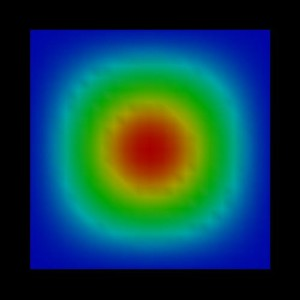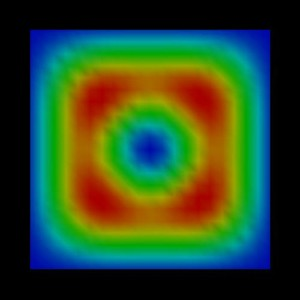1st eigenstate:` -0.0151 eV` 2nd eigenstate:``` -0.0375 eV```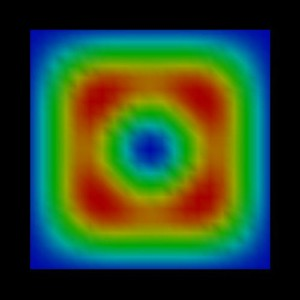3rd eigenstate:``` -0.0378 eV``` 4th eigenstate:` -0.0602 eV`

Here, the probabilty density of the second eigenstate is plotted viewed from a different perspective.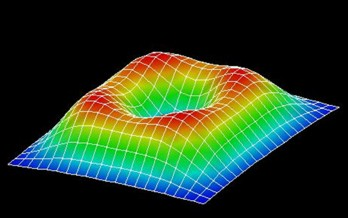Note: Currently magnetic field only works for option``` schroedinger-masses-anisotropic = yes```.

### Hole wave functions - 6-band k.p approximation (infinite GaAs barriers)

```-> 2Dwire_6x6kp.in -> 2Dwire_6x6kp_Burt.in```

The following figures show the charge densities (Psi²; Psi = hole wave function) of the four lowest energy confined hole eigenstates in an infinitely deep 10 nm x 10 nm InAs quantum wire. This time we used 6-band k.p theory to describe the hole states. Here, the second and the third eigenstate are no longer degenerate. Only the ground state looks similar to the single-band effective-mass approximation results.

We used the following Luttinger parameters for InAs:
gamma1 = 20.0, gamma2 = 8.5, gamma3 = 9.2
This corresponds to the Dresselhaus parameters L, M, N:
L = `-`55, M = `-`4, N = `-`55.2

``` 6x6kp-parameters = -55.000d0 -4.000d0 -55.200d0 ! L, M, N [hbar^2/2m](--> divide by hbar^2/2m)                      0.39d0                      ! delta_split-off [eV]  ```1st /2nd eigenstate:``` -0.0342 eV``` 3rd/4th eigenstate:``` -0.0367 eV```5th/6th eigenstate:``` -0.0557 eV``` 7th/8th eigenstate:``` -0.0581 eV```

The energies are contained in this file:``` ev_hl_6x6kp_qc001_num_kpar001_2D.dat```

``` num_ev:  eigenvalue[eV]:    cb:       hh:       lh:       s/o:  1        -0.34226968E-01    0.000E+00 0.894E-01 0.905E+00 0.519E-02  2        -0.34226968E-01    0.000E+00 0.894E-01 0.905E+00 0.519E-02  3        -0.36675499E-01    0.000E+00 0.285E+00 0.710E+00 0.528E-02  4        -0.36675499E-01    0.000E+00 0.285E+00 0.710E+00 0.528E-02  5        -0.55698284E-01    0.000E+00 0.263E+00 0.718E+00 0.192E-01  6        -0.55698284E-01    0.000E+00 0.263E+00 0.718E+00 0.192E-01  7        -0.58120024E-01    0.000E+00 0.384E+00 0.604E+00 0.113E-01  8        -0.58120024E-01    0.000E+00 0.384E+00 0.604E+00 0.113E-01```

We used:
``` schroedinger-kp-ev-solv        = ARPACK          !  schroedinger-kp-discretization = box-integration !  kp-vv-term-symmetrization      = yes             ! 'yes' (= incorrect physics), 'no' (= correct physics)```

Comment:``` schroedinger-kp-ev-solv = chearn ```leads to very similar (almost identical) results for 6-band k.p
for both options of ```kp-vv-term-symmetrization = yes/no```.

However, using instead Burt's non-symmetrized discretization```  kp-vv-term-symmetrization      = no              ! 'yes', 'no' ```leads to the following results: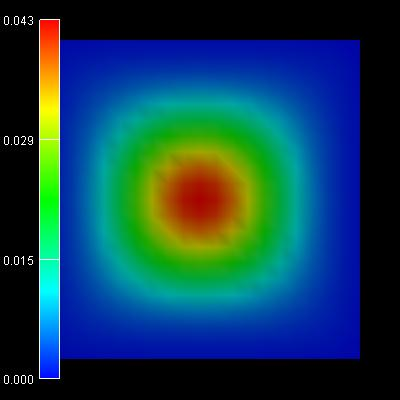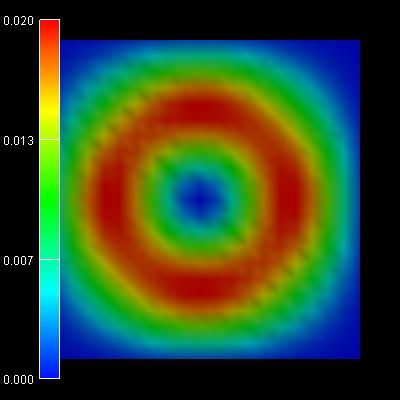1st /2nd eigenstate:``` -0.0383 eV``` 3rd/4th eigenstate:``` -0.0419 eV```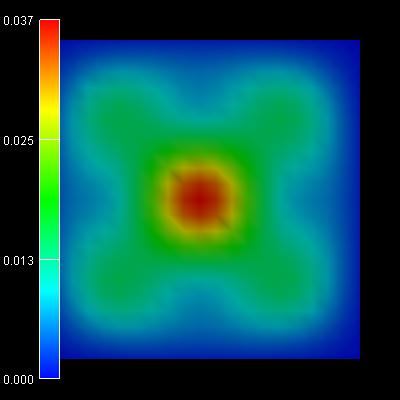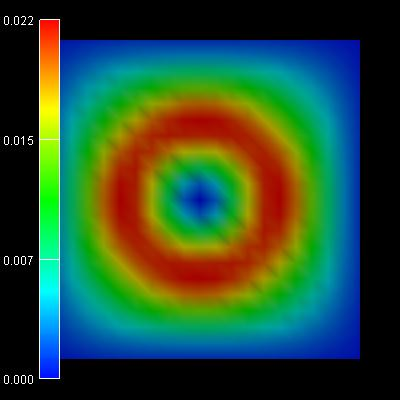5th/6th eigenstate:``` -0.0679 eV``` 7th/8th eigenstate:``` -0.0768 eV```

``` num_ev:  eigenvalue[eV]:    cb:       hh:       lh:       s/o:  1        -0.38319736E-01    0.000E+00 0.531E-01 0.941E+00 0.606E-02  2        -0.38319736E-01    0.000E+00 0.531E-01 0.941E+00 0.606E-02  3        -0.41904230E-01    0.000E+00 0.265E+00 0.735E+00 0.860E-03  4        -0.41904230E-01    0.000E+00 0.265E+00 0.735E+00 0.860E-03  5        -0.67878674E-01    0.000E+00 0.414E+00 0.584E+00 0.180E-02  6        -0.67878675E-01    0.000E+00 0.414E+00 0.584E+00 0.180E-02  7        -0.76825362E-01    0.000E+00 0.111E+00 0.874E+00 0.145E-01  8        -0.76825362E-01    0.000E+00 0.111E+00 0.874E+00 0.145E-01```

The following is obsolete because the feature``` valence-band-masses-from-kp ```does not really make much sense.

### Hole wave functions - Single-band effective-mass approximation (infinite GaAs barriers)

`-> 2Dwire_vbmassesfrom6x6kp.in`

Here we use a single-band effective-mass approximation but we use an effective-mass tensor that is obtained from the Luttinger parameters.
``` valence-band-masses-from-kp     = yes ! 'yes', 'no'  schroedinger-masses-anisotropic = yes ! 'yes', 'no', 'box'```

We used the following Luttinger parameters for InAs:
gamma1 = 20.0, gamma2 = 8.5, gamma3 = 9.2
This corresponds to the Dresselhaus parameters L, M, N:
L = `-`55, M = `-`4, N = `-`55.2

``` 6x6kp-parameters = -55.000d0 -4.000d0 -55.200d0 ! L, M, N [hbar^2/2m](--> divide by hbar^2/2m)                      0.39d0                      ! delta_split-off [eV]```

This leads to the following effective-mass tensor components for the heavy hole:
mxx =   0.333
myy =   0.333
mzz =   0.333
mxy = `-`0.943
mxz = `-`0.943
myz = `-`0.943

The following figures show the charge densities (Psi²; Psi = hole wave function) of the five lowest energy confined hole eigenstates in an infinitely deep 10 nm x 10 nm InAs quantum wire. The degeneracy of the second and the third eigenstate is now lifted.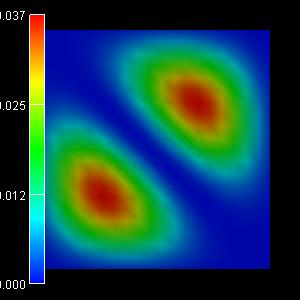1st eigenstate:``` -0.0182 eV (-0.0182)``` 2nd eigenstate:``` -0.0407 eV (-0.0406)```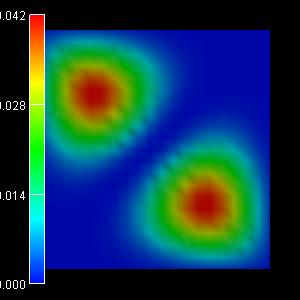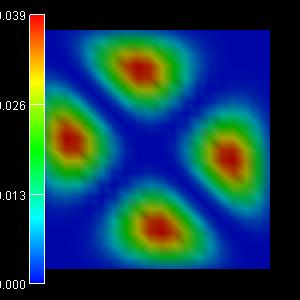3rd eigenstate:``` -0.0500 eV (-0.0500)``` 4th eigenstate:``` -0.0665 eV (-0.0662)``` 5th eigenstate:``` -0.0896 eV (-0.0895)```

In the brackets, the eigenvalues for``` schroedinger-masses-anisotropic = box ```are given.

`-> 2Dwire_vbmassesfrom6x6kp_iso.in`

``` schroedinger-masses-anisotropic = no  ! 'yes', 'no', 'box' ```then the off-diagonal elements of the effective-mass tensor are not taken into account.
mxy = `-`0.943 := 0` <- assumed to be zero!!!`
mxz = `-`0.943 := 0` <- assumed to be zero!!!`
myz = `-`0.943 := 0``` <- assumed to be zero!!! ```Due to the symmetry of the quantum wire and the effective mass tensor, the second and the third eigenstate are degenerate.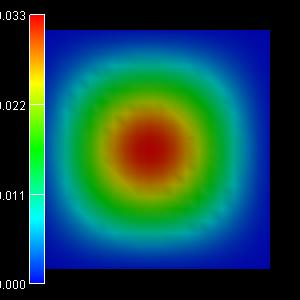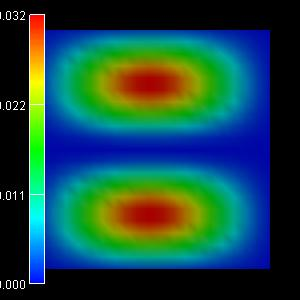1st eigenstate:` -0.0186 eV` 2nd eigenstate:` -0.0463 eV`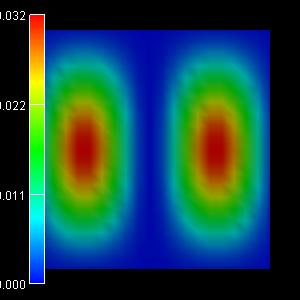3rd eigenstate:` -0.0463 eV` 4th eigenstate:` -0.0741 eV`
• Please help us to improve our tutorial. Send comments to``` support [at] nextnano.com```.